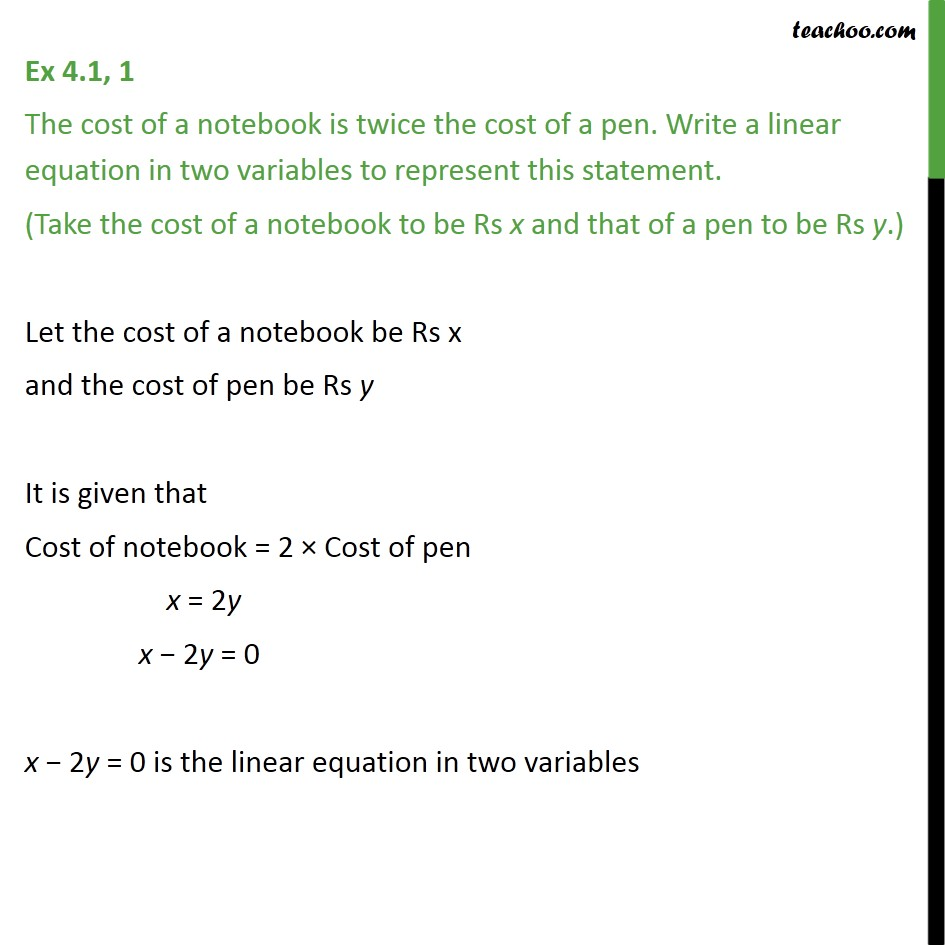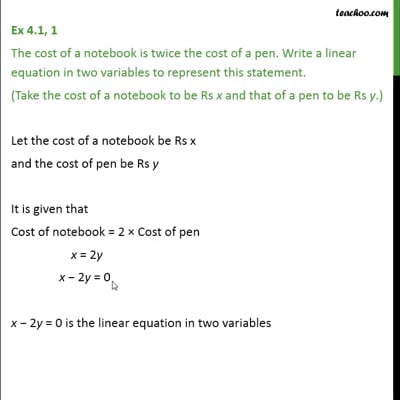Ex 4.1

Chapter 4 Class 9 Linear Equations in Two Variables
Serial order wiseThis video is only available for Teachoo black users

Learn in your speed, with individual attention - Teachoo Maths 1-on-1 Class

### Transcript

Ex 4.1, 1 The cost of a notebook is twice the cost of a pen. Write a linear equation in two variables to represent this statement. (Take the cost of a notebook to be Rs x and that of a pen to be Rs y.) Let the cost of a notebook be Rs x and the cost of pen be Rs y It is given that Cost of notebook = 2 Cost of pen x = 2y x 2y = 0 x 2y = 0 is the linear equation in two variables